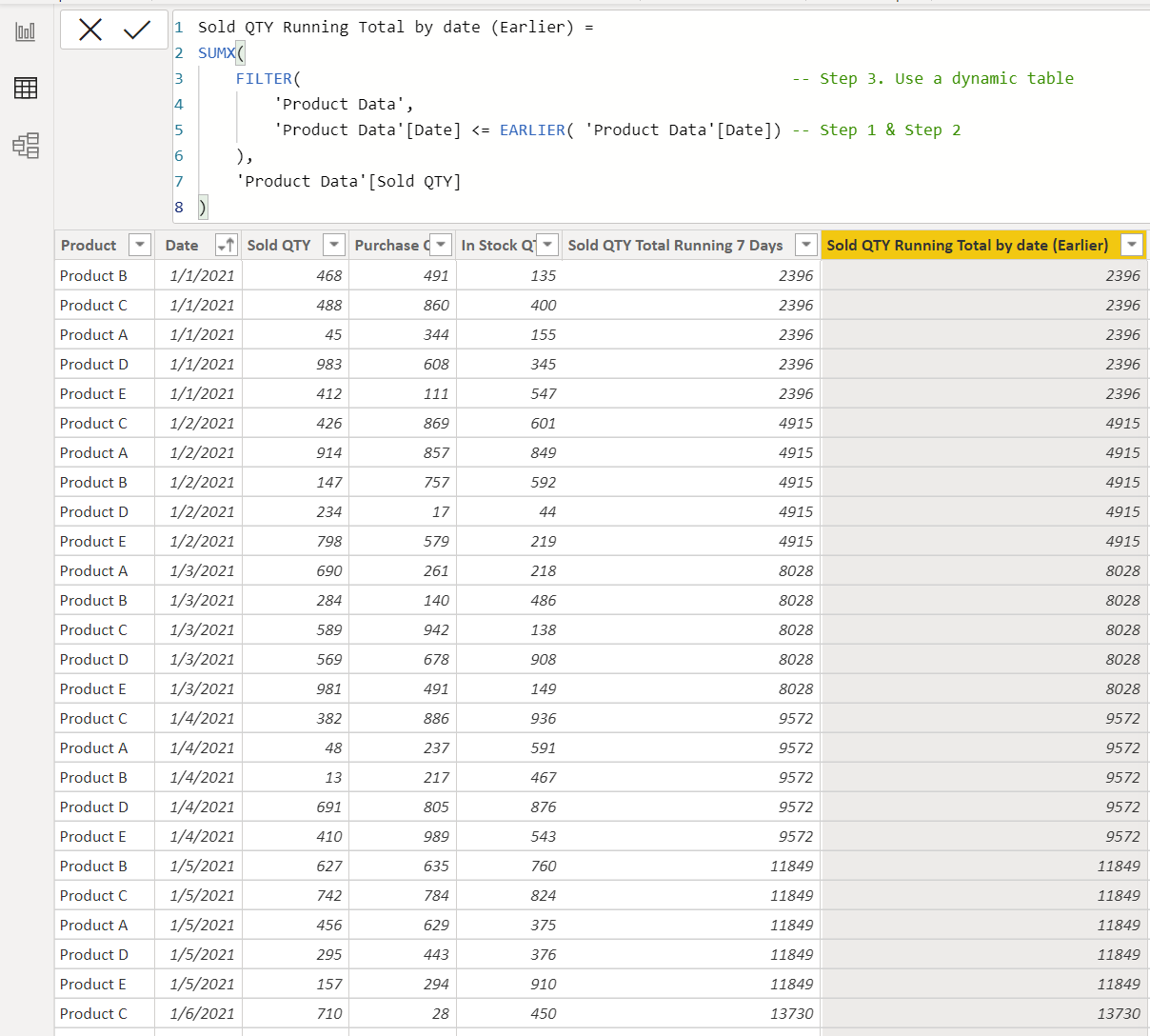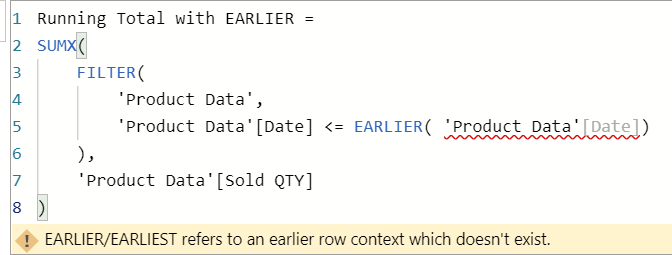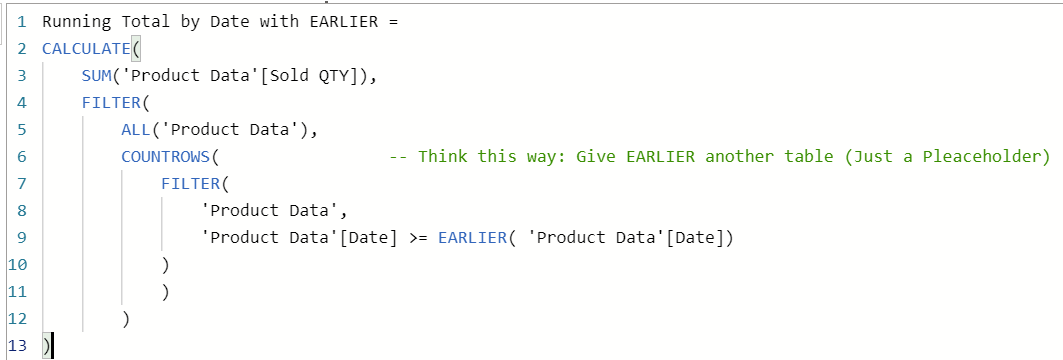# What is EARLIER?

• Syntax: EARLIER(<column>, (number))
• The purpose of the function is to create a reference to a column value on an outer loop of the evaluation of the expression.
• EARLIER is mostly used in the context of calculated columns. That is, EARLIER is commonly used in the physical tables in your data model.
• The parameter (number) is optional.

# Scenarios to use EARLIER function

Here are some situations that EARLIER would come in handy.
(1) Running Total (Yes, we discussed this in the previous article.) This is another way to execute it.
(2) How many times an item shows in a column

• Step 2. To get the running total, we need to decide if the date value of the row is earlier than the specific row.
• Step 3. Use a dynamic table with a filter condition to get the running totalEARLIER is commonly used in the Calculated Column.
• Step 2. To get the running total, we need to decide if the date value of the row is earlier than the specific row.
• Step 3. Use a dynamic table with a filter condition to get the running total
• Step 4. Use COUNTROWS to count the number of occurrences of a date

# Use EARLIER in a DAX measure

Can we use EARLIER is a DAX measure? The answer is yes! However, we seldom see EARLIER in a DAX measure. Why?The Error Message if you type an EARLIER in a measure withoutUse EARLIER in a measure

# Conclusion

EARLIER is a useful function if you are familiar with it. Here we talk about the common situations that EARLIER would be useful.

--

--